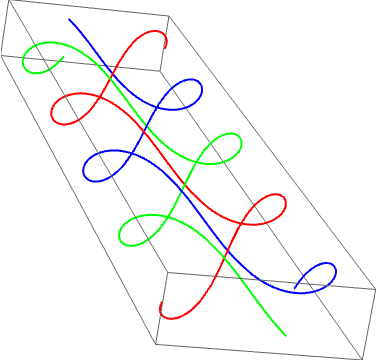# The n-body problem

With basic calculus, it’s possible to solve the problem of two masses orbiting under gravitational attraction. In particular, the only solution is for them to follow conic sections, sharing a common focus (the barycentre of the two particles). For example, Pluto and its moon Charon follow approximately circular orbits around each other, where the barycentre is closer to Pluto due to its larger mass.

### Thirteen new families of solutions

On the other hand, the three-body problem is not so simple, due to the lack of analytic solution. The simplest orbit is where three identical masses follow a circular orbit, separated by equal angles of 2π/3. Another planar solution was discovered in the 1970s. More recently, in 1993, Christopher Moore showed that they could follow a planar figure-eight path. It’s possible to add a component of the velocity perpendicular to this plane, so that the trajectories of the masses describe a braid:A few weeks ago, physicists at the Institute of Belgrade discovered a further 13 families of solutions, as reported here.

### Undecidability

The N-body problem has been proved undecidable by Warren Smith. Specifically, he carefully phrased it as a decision problem:

Given two concentric spheres in spacetime, S inside T, it is possible to convert any halting problem into a scenario where the bodies enter S if the machine halts, or remain outside T if the machine doesn’t halt (the third intermediate scenario not occurring). Hence, it is even undecidable to approximate the motion of the bodies, even for a finite length of time. Fortunately, naturally occurring orbits are sufficiently well-behaved that numerical integration suffices to predict them to a reasonable degree of accuracy.

In this tour-de-force, Warren Smith also demonstrated that relativistic and quantum-mechanical analogues of this problem are, bizarrely, computable! This is unusual, since quantum-mechanical problems are usually more difficult to compute than their classical counterparts. He concludes, therefore, that if the laws of physics are computable, this immediately rules out Newtonian mechanics without the need for experiment.

This was considered to be one of the ‘finest results’, according to the aforementioned Warren Smith.

This entry was posted in Uncategorized. Bookmark the permalink.

### 0 Responses to The n-body problem

1.Unanimous Cowherd says:

That suggests an even more roundabout argument against a Newtonian universe: note that Nick Bostrom’s simulation argument predicts that we should observe a computable universe, and therefore QM and relativity. 🙂

2.wojowu says:

http://citeseerx.ist.psu.edu/viewdoc/summary?doi=10.1.1.54.3570
You can post this link to result where Smith proves that, given initial configuration and time period, we can’t compute if within that time any singularity happens